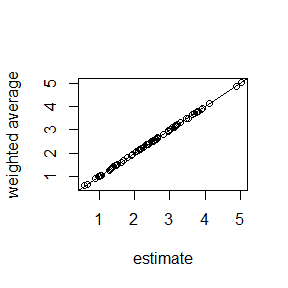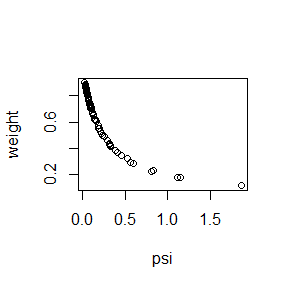# Basic area-level model

The basic area-level model (Fay and Herriot 1979; Rao and Molina 2015) is given by $y_i | \theta_i \stackrel{\mathrm{ind}}{\sim} {\cal N} (\theta_i, \psi_i) \,, \\ \theta_i = \beta' x_i + v_i \,,$ where $$i$$ runs from 1 to $$m$$, the number of areas, $$\beta$$ is a vector of regression coefficients for given covariates $$x_i$$, and $$v_i \stackrel{\mathrm{ind}}{\sim} {\cal N} (0, \sigma_v^2)$$ are independent random area effects. For each area an observation $$y_i$$ is available with given variance $$\psi_i$$.

First we generate some data according to this model:

m <- 75L  # number of areas
df <- data.frame(
area=1:m,      # area indicator
x=runif(m)     # covariate
)
v <- rnorm(m, sd=0.5)    # true area effects
theta <- 1 + 3*df$x + v # quantity of interest psi <- runif(m, 0.5, 2) / sample(1:25, m, replace=TRUE) # given variances df$y <- rnorm(m, theta, sqrt(psi))

A sampler function for a model with a regression component and a random intercept is created by

library(mcmcsae)
model <- y ~ reg(~ 1 + x, name="beta") + gen(factor = ~iid(area), name="v")
sampler <- create_sampler(model, sigma.fixed=TRUE, Q0=1/psi, linpred="fitted", data=df)

The meaning of the arguments used here is as follows:

• the first argument is a formula specifying the response variable and the linear predictor part of the model
• sigma.fixed=TRUE signifies that the observation level variance parameter is fixed at 1. In this case it means that the variances are known and given by psi.
• the function expects precisions rather than variances, and with Q0=1/psi the precisions are set to the vector 1/psi.
• linpred="fitted" indicates that we wish to obtain samples from the posterior distribution for the vector $$\theta$$ of small area means.
• data is the data.frame in which variables used in the model specification are looked up.

An MCMC simulation using this sampler function is then carried out as follows:

sim <- MCMCsim(sampler, store.all=TRUE, verbose=FALSE)

A summary of the results is obtained by

(summ <- summary(sim))
## llh_ :
##     Mean       SD  t-value     MCSE    q0.05     q0.5    q0.95    n_eff    R_hat
##  -27.958    5.617   -4.977    0.113  -37.599  -27.505  -19.285 2474.167    0.999
##
## linpred_ :
##    Mean    SD t-value    MCSE q0.05 q0.5 q0.95 n_eff R_hat
## 1  3.21 0.332    9.68 0.00609  2.67 3.21  3.75  2967 0.999
## 2  2.17 0.200   10.84 0.00365  1.84 2.17  2.50  3000 1.001
## 3  3.12 0.248   12.60 0.00471  2.73 3.13  3.52  2770 0.999
## 4  3.16 0.235   13.45 0.00443  2.76 3.17  3.53  2812 1.000
## 5  3.48 0.163   21.37 0.00304  3.21 3.48  3.75  2859 0.999
## 6  3.78 0.289   13.07 0.00529  3.32 3.78  4.25  3000 1.001
## 7  2.49 0.250    9.96 0.00456  2.07 2.48  2.90  3000 1.000
## 8  2.43 0.194   12.50 0.00354  2.11 2.43  2.74  3000 1.000
## 9  1.47 0.252    5.86 0.00467  1.07 1.47  1.90  2898 1.001
## 10 2.21 0.300    7.35 0.00610  1.72 2.20  2.69  2419 1.001
## ... 65 elements suppressed ...
##
## beta :
##              Mean    SD t-value    MCSE q0.05  q0.5 q0.95 n_eff R_hat
## (Intercept) 0.979 0.145    6.78 0.00765 0.732 0.983  1.21   357     1
## x           3.112 0.249   12.48 0.01346 2.717 3.107  3.53   343     1
##
## v_sigma :
##     Mean       SD  t-value     MCSE    q0.05     q0.5    q0.95    n_eff    R_hat
## 4.82e-01 5.88e-02 8.21e+00 1.33e-03 3.96e-01 4.78e-01 5.85e-01 1.95e+03 1.00e+00
##
## v :
##        Mean    SD t-value    MCSE   q0.05      q0.5   q0.95 n_eff R_hat
## 1   0.34996 0.332  1.0555 0.00608 -0.1915  0.342391  0.8942  2969 1.000
## 2  -0.67638 0.206 -3.2781 0.00465 -1.0160 -0.675347 -0.3420  1968 1.000
## 3   0.03780 0.252  0.1498 0.00560 -0.3717  0.034257  0.4504  2029 1.000
## 4  -0.40845 0.249 -1.6381 0.00633 -0.8251 -0.405081 -0.0109  1551 1.004
## 5   0.38716 0.180  2.1455 0.00491  0.0927  0.383639  0.6839  1352 1.002
## 6   0.12312 0.298  0.4134 0.00633 -0.3636  0.117878  0.6188  2214 0.999
## 7   0.24721 0.255  0.9679 0.00510 -0.1711  0.245228  0.6648  2509 1.000
## 8  -0.02883 0.201 -0.1431 0.00485 -0.3492 -0.024925  0.2946  1728 1.000
## 9   0.00438 0.261  0.0168 0.00637 -0.4197  0.000961  0.4469  1679 1.000
## 10 -0.54875 0.300 -1.8279 0.00625 -1.0299 -0.551127 -0.0567  2307 1.001
## ... 65 elements suppressed ...

In this example we can compare the model parameter estimates to the ‘true’ parameter values that have been used to generate the data. In the next plots we compare the estimated and ‘true’ random effects, as well as the model estimates and ‘true’ estimands. In the latter plot, the original ‘direct’ estimates are added as red triangles.

plot(v, summ$v[, "Mean"], xlab="true v", ylab="posterior mean"); abline(0, 1) plot(theta, summ$linpred_[, "Mean"], xlab="true theta", ylab="estimated"); abline(0, 1)
points(theta, df$y, col=2, pch=2)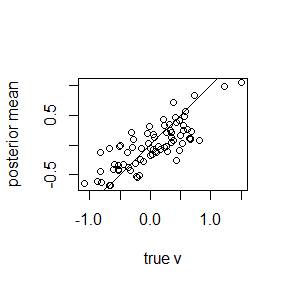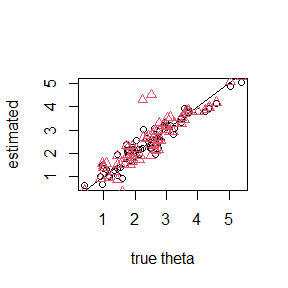We can compute model selection measures DIC and WAIC by compute_DIC(sim) ## DIC p_DIC ## 104.62721 48.71086 compute_WAIC(sim, show.progress=FALSE) ## WAIC1 p_WAIC1 WAIC2 p_WAIC2 ## 75.99048 20.06448 96.98012 30.55930 Posterior means of residuals can be extracted from the simulation output using method residuals. Here is a plot of (posterior means of) residuals against covariate $$x$$: plot(df$x, residuals(sim, mean.only=TRUE), xlab="x", ylab="residual"); abline(h=0)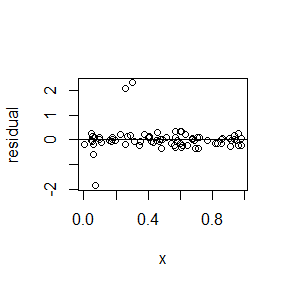A linear predictor in a linear model can be expressed as a weighted sum of the response variable. If we set compute.weights=TRUE then such weights are computed for all linear predictors specified in argument linpred. In this case it means that a set of weights is computed for each area.

sampler <- create_sampler(model, sigma.fixed=TRUE, Q0=1/psi,
linpred="fitted", data=df, compute.weights=TRUE)
sim <- MCMCsim(sampler, store.all=TRUE, verbose=FALSE)

Now the weights method returns a matrix of weights, in this case a 75 $$\times$$ 75 matrix $$w_{ij}$$ holding the weight of direct estimate $$i$$ in linear predictor $$j$$. To verify that the weights applied to the direct estimates yield the model-based estimates we plot them against each other. Also shown is a plot of the weight of the direct estimate for each area in the predictor for that same area, against the variance of the direct estimate.

plot(summ$linpred_[, "Mean"], crossprod(weights(sim), df$y),
xlab="estimate", ylab="weighted average")
abline(0, 1)
plot(psi, diag(weights(sim)), ylab="weight")